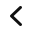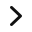# Helpful Multiplication Fluency Strategies to Boost Every Student

Teach these foundational multiplication fluency strategies so your students can have success with the multiplication facts!

In the first part of this series, I wrote that we need to teach mental math strategies for multiplication. But first, conceptual understanding of multiplication should be taught through arrays, equal groups, repeated addition, etc.. Multiplication fluency is developed alongside conceptual understanding.

From conceptual understanding, teachers can then demonstrate a multiplication fluency strategy.  At this point as well, students can begin memorizing the multiplication facts.

But, what usually happens is that some students are good at memorizing.  However,  most will learn a multiplication table and then start forgetting it soon thereafter. Yes, there are ways to improve memorization skills. I do teach my students some memorization tips.

But memorization is only ONE part of multiplication fact fluency. We also know that we will have many students who will struggle to memorize all the multiplication facts. The stress of timed tests can also interfere with memory recall.

## What do they mean by Fluency?

As this article from the National Council of Teachers of Mathematics explains, it’s complicated.

Computational fluency refers to having efficient and accurate methods for computing. Students exhibit computational fluency when they demonstrate flexibility in the computational methods they choose, understand and can explain these methods, and produce accurate answers efficiently. The computational methods that a student uses should be based on mathematical ideas that the student understands well, including the structure of the base-ten number system, properties of multiplication and division, and number relationships.

## So, What does that Mean for Multiplication Fluency?

Having flexibility in computational methods is the key to having true multiplication fact fluency.  That’s why students need to have a repertoire of multiplication fluency strategies so they don’t rely solely on memorization.

There are two categories of strategies:  Foundational Strategies and Derivative Strategies. These multiplication fluency strategies are to be practiced with paper and pencil first, but eventually (just like the addition strategies) they begin to use them mentally with more efficiency.

## What are the Foundation Strategies for Multiplication Fact Fluency?

The Foundation Strategies must be taught first as they are the base for the later Derivative Strategies.

The Foundation Strategies involve basic mental math skills such as

• finding a pattern (related to skip-counting)
• knowing the Identity and Zero Properties of Multiplication
• and learning by memory the all-important products square numbers (6×6 = 36, etc.).

Here’s a chart comparing the Foundation Strategies for both Addition and Multiplication.

If a student uses these multiplication fact strategies, the student will know about 50 facts on a multiplication chart!  That is a great start to eventually learning the rest and being fluent in the computation of multiplication facts.  The student must have these skills under control in order to advance to the next level of strategies, the Derivative Strategies.

## How to Teach the Foundation Strategies for Multiplication Fact Fluency

Skip counting is one of those mental math skills that begins early in kindergarten.  By second grade children should be able to count by 2s, 5s, and 10s.  Counting by 2s, 5s, and 10s will enable students to quickly learn the multiplication facts for those tables.  It also helps them internalize the patterns found in multiplication.

Skip counting can be done with songs (https://youtu.be/SCBwSSDk9Mg).  They are a great way to motivate kids and keep them engaged while learning.  Counting coins such as dimes and nickels is another way to motivate students to learn to count by 5s and 10s (and see the patterns!).

A game for learning to count by 2s is thinking of things that come in pairs.

• How many pairs of eyes are in our class?
• How many shoes?
• There are lots of possibilities!
• But also games, flashcards, multiples strips, and bookmarks also help with learning to count by 2s, 5s, and 10s.

I’ve made some of my own games and resources for students to become fluent with these facts. They are part of a complete resource for teaching all the multiplication fluency strategies.

## The Properties of Multiplication

Teaching and learning the Properties of Multiplication lay the foundation later for Algebra and more advanced math.  So it is important that we explicitly teach these building block properties.  Start with the Identity Property and the Zero Property.

I like to teach these two properties in a fun way by making Zero a Hero and One a Bum.  Zero can obliterate any factor to produce a product of zero while One is a bum because he’s lazy and produces a product equivalent to the factor.

Most students will acquire this multiplication strategy quickly, though they might confuse the names of the properties.  The Common Core State Standards for Mathematics in Grade 3 states that students do not need to use the formal terms of the properties. However, using precise language is part of the Standards for Mathematical Practice (SMP6).

## Another Strategy is Learning the Multiplication Squares

Finally, the products of squares (2 x 2, 3 x 3, 4 x 4, etc.) should be learned and memorized.  The square numbers run diagonally across the multiplication chart.  The resulting line of squares produces a mirror image of the other products (3 x 4 = 12 and 4 x 3 = 12).

That is why it’s important to memorize the products of square numbers.  There are only 10 square product facts to memorize. Overall, it reduces the number of facts to learn!

To teach the square numbers is to make cards with all the square factors (1 x 1, 2 x 2, 3 x 3, etc) on one card.  Then on other cards write all the products of those squares.

• Then hand out one card to each student.
• On a signal, they are to quietly get up and find their partner to match the square factors to the square product.
• When they’ve found their match, the pair stands back to back.
• You can practice this multiple times in a 5 – 10 minute period by giving students a different card each time.
• You can even time the class to see how fast all the pairs can partner up.

Students should practice the Foundation Strategies until they are proficient because they will be needed later to develop the Derivative Strategies.

## What Comes Next in Multiplication Fluency?

In Part 3, we’ll take a look at the Derivative Examples including a video of one strategy used in action!

What are some of your best ways of teaching skip counting? The Properties of Multiplication? Please share your ideas in the comments below!

In the meantime, check out the latest resource in my Teachers Pay Teachers store!  Made explicitly for teaching multiplication fluency strategies!Teaching Posters
Derivative Strategies for Multiplication
Commutative Property
Multiplication Fluency Games
Practice Templates
Practice Pages
Teacher Support## Don’t Go Yet!

Are you eager to try some of these strategies with your students right now? I’m offering this FREE Guide to Achieving Multiplication Fluency!

It’s a 15-page guide that explains all the strategies.  It has some suggestions for teaching these strategies and how to assess strategy use.  It’s a comprehensive guide to boost student fluency with the multiplication facts. It is not a miracle cure and does not guarantee instant fluency. What it does is give your students strategies for achieving that fluency by the end of a school year.

It’s FREE and you can get it by subscribing to my newsletter.

What other effective strategies do you use to teach fact fluency?1.